×#### Thank you for registering.

One of our academic counsellors will contact you within 1 working day.

Click to Chat

1800-1023-196

+91-120-4616500

CART 0

• 0

MY CART (5)

Use Coupon: CART20 and get 20% off on all online Study Material

ITEM
DETAILS
MRP
DISCOUNT
FINAL PRICE
Total Price: Rs.

There are no items in this cart.
Continue Shopping• Complete JEE Main/Advanced Course and Test Series
• OFFERED PRICE: Rs. 15,900
• View Details

```Chapter 14: Quadratic Equations – Exercise 14.1

Quadratic Equations – Exercise 14.1 – Q.1

x2 + 1 = 0

⟹ x2 + i2 = 0                [∵ i2 = – 1]

⟹ (x + i)(x - i) = 0       [a2 - b2 = (a + b)(a - b)]

⟹ x = i, -i

Quadratic Equations – Exercise 14.1 – Q.2

9x2 + 4 = 0

⟹(3x)2 - (2i)2 = 0              [∵ i2 = – 1]

⟹ (3x + 2i)(3x – 2i) = 0

⟹ 3x + 2i = 0 or 3x = 2i = 0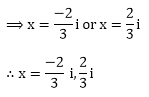Quadratic Equations – Exercise 14.1 – Q.3

x2 + 2x + 5 = 0

Now, completing the squares, we get

(x + 1)2 + 4 = 0

⟹ (x + 1)2 - 2i2 = 0

⟹ (x + 1 + 2i)(x + 1 - 2i) = 0

⟹ (x + 1 + 2i) = 0 or (x + 1 - 2i) = 0

∴ x = – 1 – 2i, – 1 + 2i

Quadratic Equations – Exercise 14.1 – Q.4

4x2 - 12x + 25 = 0

⟹ (2x – 3)2 - 4i2 = 0

⟹ (2x – 3 + 4i) (2x – 3 - 4i) = 0

⟹ (2x – 3 + 4i) = 0 or (2x – 3 – 4i) = 0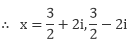Quadratic Equations – Exercise 14.1 – Q.5

x2 + x + 1 = 0

Now, completing the squares, we get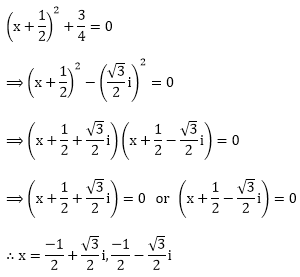Quadratic Equations – Exercise 14.1 – Q.6

4x2 + 1 = 0

⟹(2x)2 - i2 = 0 [ ∵ i2 = – 1]

⟹(2x + i)(2x – i) = 0

⟹ either 2x + i = 0 or 2x – i = 0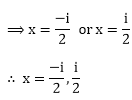Quadratic Equations – Exercise 14.1 – Q.7

x2 - 4x + 7 = 0

We will apply discriminant rule,Where D = b2 - 4ac =(-4)2 - 4.1.7 = – 12

from (A)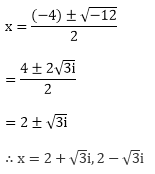Quadratic Equations – Exercise 14.1 – Q.8

x2 + 2x + 2 = 0

We will apply discriminant rule,Where D = b2 - 4ac

= 22 - 4.1.2

= 4 – 8

= – 4

Quadratic Equations – Exercise 14.1 – Q.9

5x2 - 6x + 2 = 0

We will apply discriminant rule,Where D = b2 - 4ac

=(-b)2 - 4.5.2

= 36 – 40

= – 4

from (A)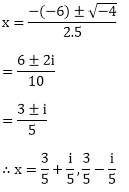Quadratic Equations – Exercise 14.1 – Q.10

21x2+ 9x + 1 = 0

Comparing the given equation with the general form

ax2 + bx + c = 0, we get a = 21, b = 9, c = 1

Substituting a and b in,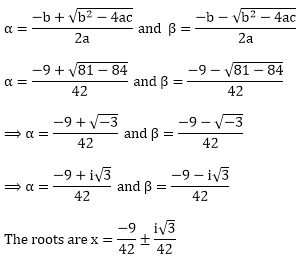Quadratic Equations – Exercise 14.1 – Q.11

x2 - x + 1 = 0

We will apply discriminant rule,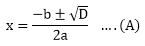Where D = b2 - 4ac

=(-1)2 - 4.1.1

=1 – 4

= – 3

from (A)Quadratic Equations – Exercise 14.1 – Q.12

x2 + x + 1 = 0

We will apply discriminant rule,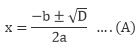Where D = b2 - 4ac

=12 - 4.1.1

=1 – 4

= – 3

from (A)Quadratic Equations – Exercise 14.1 – Q.13

17x2 - 8x + 1 = 0

We will apply discriminant rule,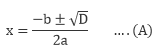Where D = b2 - 4ac

=(-8)2 - 4.17.1

= 64 – 68

= – 4

from (A)Quadratic Equations – Exercise 14.1 – Q.14

27x2 - 10x + 1 = 0

We will apply discriminant rule,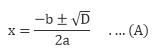Where D = b2 - 4ac

= (-10)2- 4.27.1

=100 – 108

= – 8

from (A)```### Course Features

• 728 Video Lectures
• Revision Notes
• Previous Year Papers
• Mind Map
• Study Planner
• NCERT Solutions
• Discussion Forum
• Test paper with Video Solution